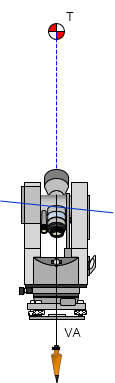### e. Horizontal Axis

#### (1) Description

The LOS is constrained to rotate around the HA. If the HA is not horizontal when the instrument is level, the LOS will not travel in a vertical plane. This will affect vertical and horizontal angle measurements.

#### (2) Check

A distinct sight mark at least 30° above horizontal is needed. A wall works well because two additional marks will be set.

Set up the instrument in the D position, Figure G-30(a).
The HA will be inclined if it is not perpendicular to the VA.
Sight the mark, T.  Rotate the telescope to approximately horizontal and set a mark, A, Figure G-30(b).(a) HA Orientation (b) Set point A Figure G-30 HA Check Direct Position

If the HA is maladjusted, the LOS will not follow a vertical path from T to A.

Reverse the instrument to the R position. This will incline the HA in the opposite direction, Figure G-31(a).
Sight the mark, T. Rotate the telescope to approximately horizontal and set a mark, B, Figure G-31(b)(A) HA Orientation (b) Set point B Figure G-31 HA Check Reverse Position

As in the D position, if the HA is maladjusted, the LOS will not follow a vertical path from T to B.

If the instrument is in adjustment, points A and B will coincide. Point C vertically below point T is halfway between points A and B, Figure G-32.Figure G-32 Vertical Point

#### (3) Compensation

##### (a) Procedure

Modern TSIs have dual-axis compensation to account for horizontal and vertical tilts. Refer to the instrument's Operation Manual for the compensation range and how to check.
Although they provide a degree of self-correction, procedural compensations should still be used with TSIs.

##### i. Vertical Angle Measurement

The maladjustment cannot be compensated by procedure for a theodolite. In both D and R positions, the vertical angle is inclined so its magnitude is always too large.

To set a point vertically below another, use the check method and set the point as shown in Figure G-32.

##### ii. Horizontal Angle Measurement

If the bascksight (BS) and foresight (FS) points are at the same vertical angle, the maladjustment will not introduce an error. However, if one point is higher or lower than the other, an error may occur if the LOS must be vertically rotated.

To measure a horizontal angle,
Measure the angle in the D position, Figure G-33.Figure G-33 Horizontal Angle Direct

Measure the angle in the R position, Figure G-34.Figure G-34 Horizontal Angle Reverse

The correct angle is the average of the D and R angles, Figure G-35.Figure G-35 Corrected Horizontal Angle